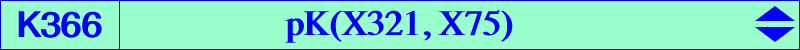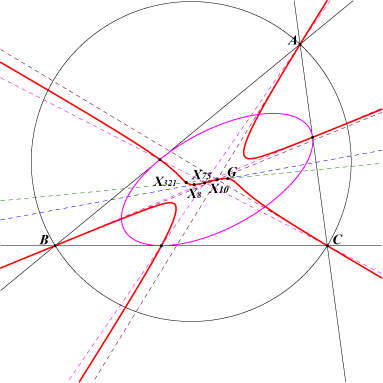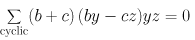X(2), X(8), X(10), X(75), X(307), X(318), X(321), X(1441)K366 is a member of the class CL042. It has the same asymptotic directions as pK(X2, X58). It is the isotomic transform of K317 = pK(X81, X86). The cevian triangle of any point on K366 is perspective with the outer Garcia triangle (see ETC, preamble of X5587) and the perspector lies on K033. See also K233. K366 is the locus of pseudo-pivots of central psKs with center X(10). The locus of pseudo-poles is K1311 = pK(X762, X10) and the locus of pseudo-isopivots is K1312 = pK(X1500, X10). One of these cubics is K033 and all four cubics are equivalent to K002. See Table 21.